Documentation

# bodeplot

Plot Bode frequency response with additional plot customization options

## Syntax

```h = bodeplot(sys) bodeplot(sys) bodeplot(sys1,sys2,...) bodeplot(AX,...) bodeplot(..., plotoptions) bodeplot(sys,w) ```

## Description

`h = bodeplot(sys)` plot the Bode magnitude and phase of the dynamic system model `sys` and returns the plot handle `h` to the plot. You can use this handle to customize the plot with the `getoptions` and `setoptions` commands.

`bodeplot(sys)` draws the Bode plot of the model `sys`. The frequency range and number of points are chosen automatically.

`bodeplot(sys1,sys2,...)` graphs the Bode response of multiple models `sys1`,`sys2`,... on a single plot. You can specify a color, line style, and marker for each model, as in

```bodeplot(sys1,'r',sys2,'y--',sys3,'gx') ```

`bodeplot(AX,...)` plots into the axes with handle `AX`.

`bodeplot(..., plotoptions)` plots the Bode response with the options specified in `plotoptions`. Type

```help bodeoptions ```

for a list of available plot options. See Match Phase at Specified Frequency for an example of phase matching using the `PhaseMatchingFreq` and `PhaseMatchingValue` options.

`bodeplot(sys,w)` draws the Bode plot for frequencies specified by `w`. When `w = {wmin,wmax}`, the Bode plot is drawn for frequencies between `wmin` and `wmax` (in `rad/TimeUnit`, where `TimeUnit` is the time units of the input dynamic system, specified in the `TimeUnit` property of `sys`.). When `w` is a user-supplied vector `w` of frequencies, in rad/TimeUnit, the Bode response is drawn for the specified frequencies.

See `logspace` to generate logarithmically spaced frequency vectors.

## Examples

collapse all

Generate a Bode plot.

```sys = rss(5); h = bodeplot(sys);```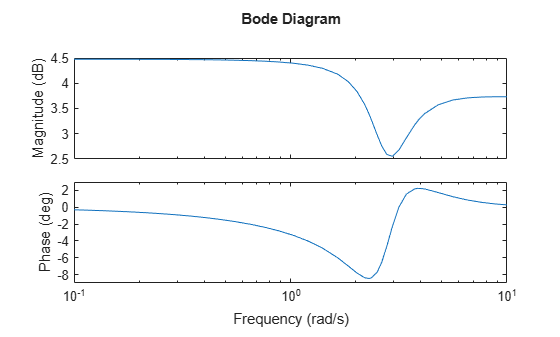Change the units to Hz and suppress the phase plot. To do so, edit properties of the plot handle, `h`.

`setoptions(h,'FreqUnits','Hz','PhaseVisible','off');`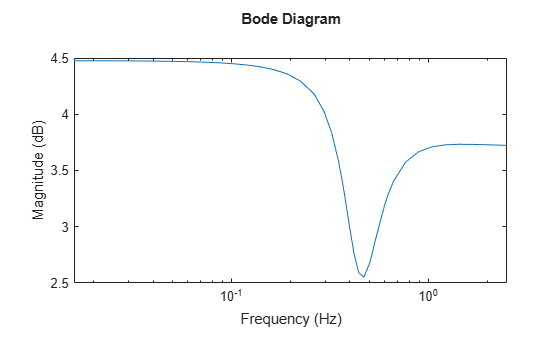The plot automatically updates when you call `setoptions`.

Create a Bode plot of a dynamic system.

```sys = tf(1,[1 1]); h = bodeplot(sys);```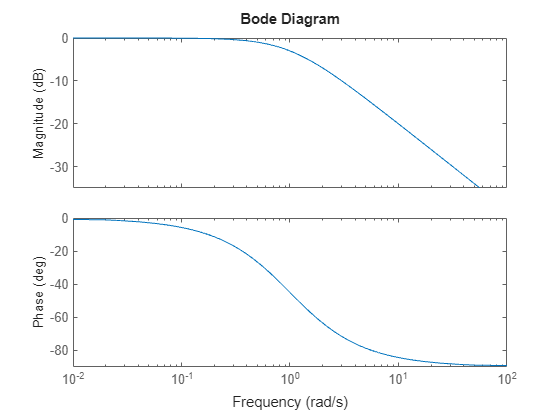Fix the phase at 1 rad/s to 750 degrees. To do so, get the plot properties. Then alter the properties `PhaseMatchingFreq` and `PhaseMatchingValue` to match a phase to a specified frequency.

```p = getoptions(h); p.PhaseMatching = 'on'; p.PhaseMatchingFreq = 1; p.PhaseMatchingValue = 750;```

Update the plot.

`setoptions(h,p);`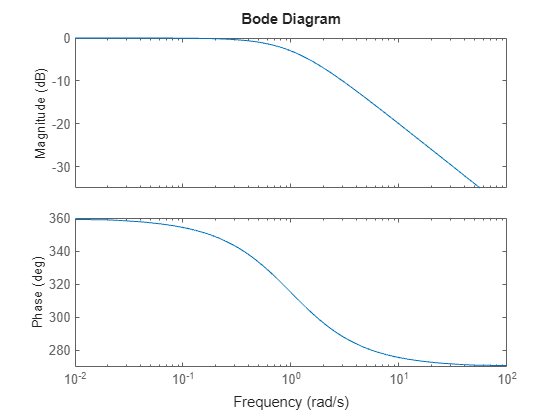The first bode plot has a phase of -45 degrees at a frequency of 1 rad/s. Setting the phase matching options so that at 1 rad/s the phase is near 750 degrees yields the second Bode plot. Note that, however, the phase can only be -45 + N*360, where N is an integer, and so the plot is set to the nearest allowable phase, namely 675 degrees (or 2*360 - 45 = 675).

Compare the frequency responses of identified state-space models of order 2 and 6 along with their 2 $\sigma$ confidence regions.

```load iddata1 sys1 = n4sid(z1, 2); sys2 = n4sid(z1, 6);```

Both models produce about 70% fit to data. However, `sys2` shows higher uncertainty in its frequency response, especially close to Nyquist frequency as shown by the plot:

```w = linspace(8,10*pi,256); h = bodeplot(sys1,sys2,w); setoptions(h,'PhaseMatching','on','ConfidenceRegionNumberSD',2);```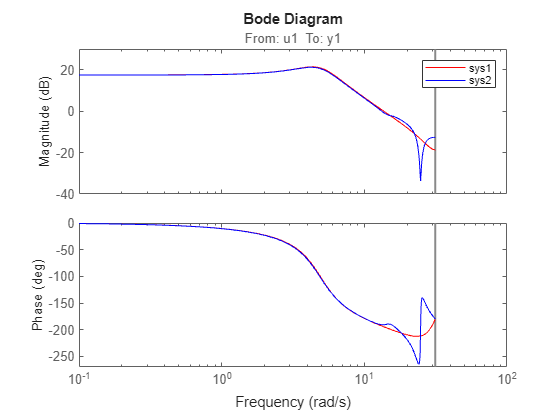Right-click the plot and select Characteristics > Confidence Region to turn on the confidence region characteristic. Alternatively, type `showConfidence(h)` to plot the confidence region.

Compare the frequency response of a parametric model, identified from input/output data, to a nonparametric model identified using the same data. Identify parametric and non-parametric models based on data.

```load iddata2 z2; w = linspace(0,10*pi,128); sys_np = spa(z2,[],w); sys_p = tfest(z2,2);```

`spa` and `tfest` require System Identification Toolbox™ software. `sys_np` is a nonparametric identified model. `sys_p` is a parametric identified model.

Create a Bode plot that includes both systems.

```opt = bodeoptions; opt.PhaseMatching = 'on'; bodeplot(sys_np,sys_p,w,opt);```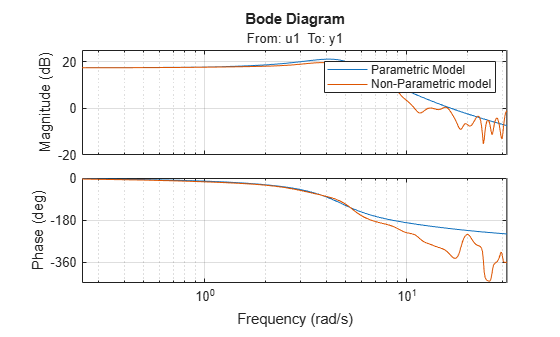## Tips

You can change the properties of your plot, for example the units. For information on the ways to change properties of your plots, see Ways to Customize Plots.

## See Also

#### Learn how to automatically tune PID controller gains

Download code examples